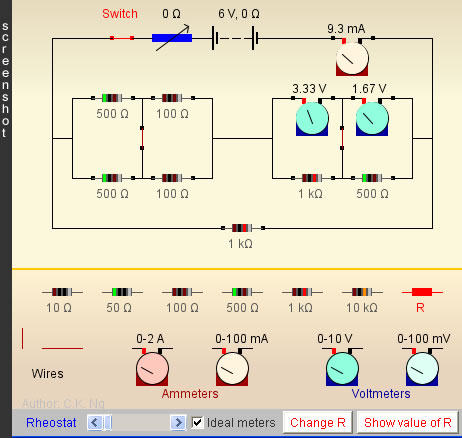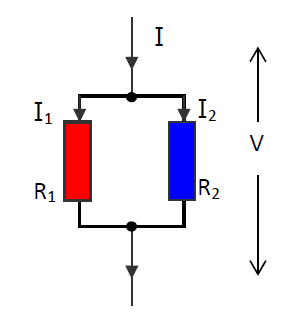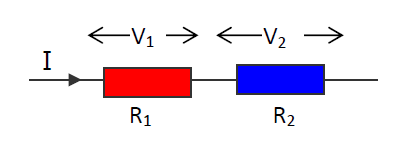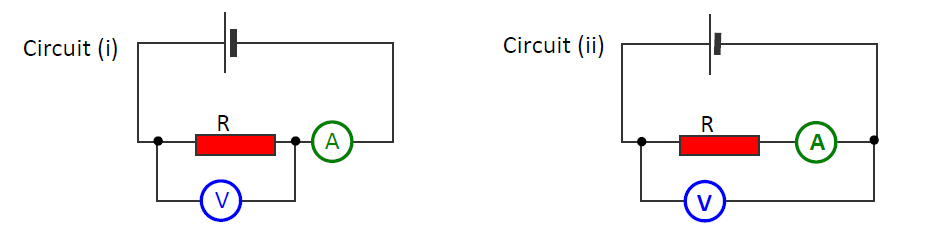Simple Electric Circuit

• Click the switch to close or open it
• Select an appropriate electric component (resistor, wire or meter) from the lower portion, click and drag it to a vacant site in the circuit. The component will fill into the vacant site when the mouse button is released.
• To remove an inserted component in the circuit, just drag it out and release.
• The resistance of the rheostat can be varied over the range 0 Ω - 100 Ω.
• Ideal ammeters and voltemters have zero and infinite internal resistances respectively. However, this assumption can be lifted, by unchecking the box "Ideal meters", then their internal resistances become 20 Ω and 10 kΩ respectively.
• The resistance of the red resistor R is not shown (unless "Show Value of R" is pressed); it could be used, e.g., in a circuit of finding an unknown resistor.
• Press "Change R" to reassign a value between 10 Ω and 10 kΩ to R randomly.
• Press " Show Value of R" to show the value of R. Press the label again to hide the value.

 Internal Links : Electric Meter (shunts and multipliers)

## Resistors in Parallel• The voltages across resistors connected in parallel are the same, but their currents are in the same ratio of the reciprocals of their resistances.
• Equivalent R: 1/R = 1/R1 + 1/R2 + 1/R3 + …
• The equivalent resistance R is smaller than the smallest among the resistors connected in parallel.
• The equivalent resistance of n identical resistors of each R is R/n.

## Resistors in Series• In series, the current passing through each resistor is the same while the voltages across them are in the same ratio of their resistances.
• Equivalent resistance: R = R1 + R2 + R3 + …
• The equivalent resistance R is larger than the largest among the resistors connected in series.
• The equivalent resistance of n identical resistors of each R is nR.

## Measuring Unknown Resistance by Voltmeter-Ammeter Method• R is measured by the formula R = voltmeter reading ÷ ammeter reading ……(*)
• Using (*), the above two circuits give two different values of R unless both the voltmeter and ammeter are ideal, i.e., the former has infinite internal resistance and the latter has zero internal resistance.
• In Circuit (i), the result of “voltmeter reading ÷ ammeter reading” is the total resistance of the parallel-combination of R and the voltmeter. But if R << internal resistance of voltmeter, their parallel-combined-resistance is about the same as R. In other words, Circuit (i) is good for measuring an unknown R of small resistance.
• In Circuit (ii), the result of “voltmeter reading ÷ ammeter reading” is the total resistance of the series-combination of R and the ammeter. But if R >> internal resistance of ammeter, their series-combined-resistance is about the same as R. In other words, Circuit (ii) is good for measuring an unknown R of large resistance.

 The author (Chiu-king Ng) has the copyright on all the simulations in this website. Email phyAA@phy.hk, where AA is the prime number following 7. Last Update:2019-9-15